Tamil Nadu Board of Secondary EducationSSLC (English Medium) Class 8th

# Tamil Nadu Board Samacheer Kalvi solutions for Class 8th Mathematics Answers Guide chapter 2 - Measurements [Latest edition]

#### Chapters## Chapter 2: Measurements

Exercise 2.1Exercise 2.2Exercise 2.3Exercise 2.4
Exercise 2.1 [Pages 58 - 59]

### Tamil Nadu Board Samacheer Kalvi solutions for Class 8th Mathematics Answers Guide Chapter 2 MeasurementsExercise 2.1 [Pages 58 - 59]

#### Fill in the blanks:

Exercise 2.1 | Q 1. (i) | Page 58

The ratio between the circumference and diameter of any circle is _______

Exercise 2.1 | Q 1. (ii) | Page 58

A line segment which joins any two points on a circle is a ___________

Exercise 2.1 | Q 1. (iii) | Page 58

The longest chord of a circle is __________

Exercise 2.1 | Q 1. (iv) | Page 58

The radius of a circle of diameter 24 cm is _______

Exercise 2.1 | Q 1. (v) | Page 58

A part of circumference of a circle is called as _______

Exercise 2.1 | Q 2 | Page 58

Match the following:

 Column A Column B (i) Area of a circle (a) 1/4 pi"r"^2 (ii) Circumference of a circle (b) (π + 2)r (iii) Area of the sector of a circle (c) πr2 (iv) Circumference of a semicircle (d) 2πr (v) Area of a quadrant of a circle (e) theta^circ/360^circ xx pi"r"^2
Exercise 2.1 | Q 3 | Page 58

Find the central angle of the shaded sectors (each circle is divided into equal sectors).

 Sectors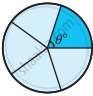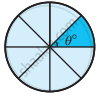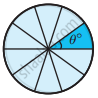Central angle of each sector (θ°)
Exercise 2.1 | Q 4. (i) | Page 58

For the sectors with given measures, find the length of the arc, area and perimeter. (π = 3.14)

central angle 45°, r = 16 cm

Exercise 2.1 | Q 4. (ii) | Page 58

For the sectors with given measures, find the length of the arc, area and perimeter. (π = 3.14)

central angle 120°, d = 12.6 cm

Exercise 2.1 | Q 5. (i) | Page 58

From the measures given below, find the area of the sectors.

Length of the arc = 48 m, r = 10 m

Exercise 2.1 | Q 5. (ii) | Page 58

From the measures given below, find the area of the sectors.

length of the arc = 50 cm, r = 13.5 cm

Exercise 2.1 | Q 6. (i) | Page 59

Find the central angle of the sectors whose measures are given below. (π = 22/7)

area = 462 cm2, r = 21 cm

Exercise 2.1 | Q 6. (ii) | Page 59

Find the central angle of the sectors whose measures are given below. (π = 22/7)

length of the arc = 44 m, r = 35 m

Exercise 2.1 | Q 7 | Page 59

A circle of radius 120 m is divided into 8 equal sectors. Find the length of the arc of each of the sectors

Exercise 2.1 | Q 8 | Page 59

A circle of radius 70 cm is divided into 5 equal sectors. Find the area of each of the sectors

Exercise 2.1 | Q 9 | Page 59

Dhamu fixes a square tile of 30 cm on the floor. The tile has a sector design on it as shown in the figure. Find the area of the sector. (π = 3.14)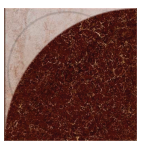Exercise 2.1 | Q 10 | Page 59

A circle is formed with 8 equal granite stones as shown in the figure each of radius 56 cm and whose central angle is 45°. Find the area of the granite stones. (pi = 22/7)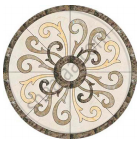Exercise 2.2 [Pages 65 - 66]

### Tamil Nadu Board Samacheer Kalvi solutions for Class 8th Mathematics Answers Guide Chapter 2 MeasurementsExercise 2.2 [Pages 65 - 66]

Exercise 2.2 | Q 1. (i) | Page 65

Find the perimeter and area of the figure given below. (pi = 22/7)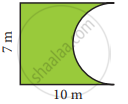Exercise 2.2 | Q 1. (ii) | Page 65

Find the perimeter and area of the figure given below. (pi = 22/7)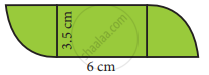Exercise 2.2 | Q 2. (i) | Page 66

Find the area of the shaded part in the following figure. (π = 3.14)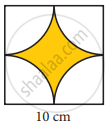Exercise 2.2 | Q 2. (ii) | Page 66

Find the area of the shaded part in the following figure. (π = 3.14)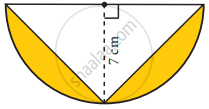Exercise 2.2 | Q 3 | Page 66

Find the area of the combined figure given which is got by joining of two parallelograms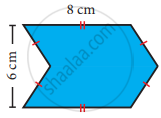Exercise 2.2 | Q 4 | Page 66

Find the area of the combined figure given, formed by joining a semicircle of diameter 6 cm with a triangle of base 6 cm and height 9 cm. (π = 3.14)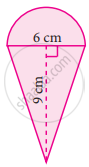Exercise 2.2 | Q 5 | Page 66

The doormat which is in a hexagonal shape has the following measures as given in the figure. Find its area.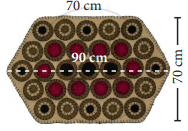Exercise 2.2 | Q 6 | Page 66

A rocket drawing has the measures as given in the figure. Find its area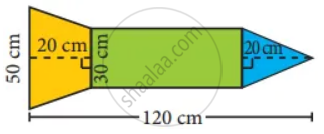Exercise 2.2 | Q 7 | Page 66

Find the area of the irregular polygon shaped fields given below.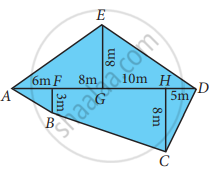Exercise 2.3 [Pages 71 - 72]

### Tamil Nadu Board Samacheer Kalvi solutions for Class 8th Mathematics Answers Guide Chapter 2 MeasurementsExercise 2.3 [Pages 71 - 72]

#### Fill in the blanks:

Exercise 2.3 | Q 1. (i) | Page 71

The three dimensions of a cuboid are ____, _____ and ____

Exercise 2.3 | Q 1. (ii) | Page 71

The meeting point of more than two edges in a polyhedron is called as ______

Exercise 2.3 | Q 1. (iii) | Page 71

A cube has __________ faces

Exercise 2.3 | Q 1. (iv) | Page 71

The cross section of a solid cylinder is __________

Exercise 2.3 | Q 1. (v) | Page 71

If a net of a 3-D shape has six plane squares, then it is called ______

#### Match the following:

Exercise 2.3 | Q 2 | Page 71

Match the following:

 Column A Column B (i)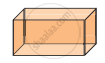(a) Cylinder (ii)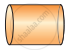(b) Cuboid (iii)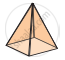(c) Triangular Prism (iv)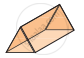(d) Square Pyramid
Exercise 2.3 | Q 3. (i) | Page 71

Which 3-D shapes do the following nets represent? Draw them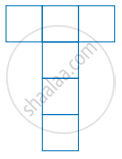Exercise 2.3 | Q 3. (ii) | Page 71

Which 3-D shapes do the following nets represent? Draw them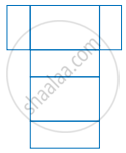Exercise 2.3 | Q 3. (iii) | Page 71

Which 3-D shapes do the following nets represent? Draw them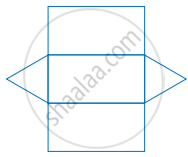Exercise 2.3 | Q 3. (iv) | Page 71

Which 3-D shapes do the following nets represent? Draw them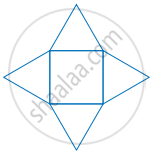Exercise 2.3 | Q 3. (v) | Page 71

Which 3-D shapes do the following nets represent? Draw them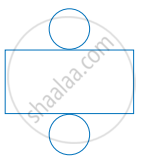Exercise 2.3 | Q 4 | Page 71

For each solid, three views are given. Identify for each solid, the corresponding Top, Front and Side (T, F and S) views.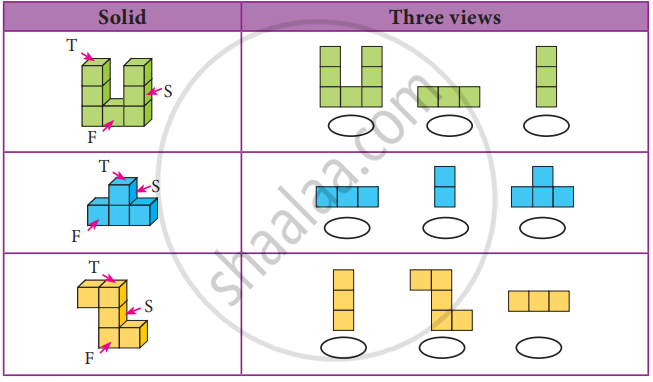Exercise 2.3 | Q 5. (i) | Page 72

Verify Euler’s formula for the table given below.

 Faces Verticles Edges 4 4 6
Exercise 2.3 | Q 5. (ii) | Page 72

Verify Euler’s formula for the table given below.

 Faces Verticles Edges 10 6 12
Exercise 2.3 | Q 5. (iii) | Page 72

Verify Euler’s formula for the table given below.

 Faces Verticles Edges 12 20 30
Exercise 2.3 | Q 5. (iv) | Page 72

Verify Euler’s formula for the table given below.

 Faces Vertices Edges 20 13 30
Exercise 2.3 | Q 5. (v) | Page 72

Verify Euler’s formula for the table given below.

 Faces Vertices Edges 32 60 90
Exercise 2.4 [Pages 72 - 73]

### Tamil Nadu Board Samacheer Kalvi solutions for Class 8th Mathematics Answers Guide Chapter 2 MeasurementsExercise 2.4 [Pages 72 - 73]

#### Miscellaneous Practice Problems

Exercise 2.4 | Q 1 | Page 72

Two gates are fitted at the entrance of a library. To open the gates easily, a wheel is fixed at 6 feet distance from the wall to which the gate is fixed. If one of the gates is opened to 90°, find the distance moved by the wheel (π = 3.14)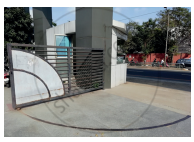Exercise 2.4 | Q 2 | Page 72

With his usual speed, if a person covers a circular track of radius 150 m in 9 minutes, find the distance that he covers in 3 minutes (π = 3.14)

Exercise 2.4 | Q 3 | Page 72

Find the area of the house drawing given in the figure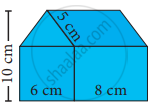Exercise 2.4 | Q 4. (i) | Page 72

Draw the top, front and side view of the following solid shapes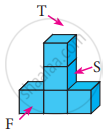Exercise 2.4 | Q 4. (ii) | Page 72

Draw the top, front and side view of the following solid shapes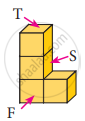#### Challenging problems

Exercise 2.4 | Q 5 | Page 73

Guna has fixed a single door of width 3 feet in his room where as Nathan has fixed a double door, each of width 1 1/2 feet in his room. From the closed position, if each of the single and double doors can open up to 120°, whose door takes a minimum area?

Exercise 2.4 | Q 6 | Page 72

In a rectangular field which measures 15 m × 8m, cows are tied with a rope of length 3 m at four corners of the field and also at the centre. Find the area of the field where none of the cow can graze. (π = 3.14)

Exercise 2.4 | Q 7 | Page 72

Three identical coins, each of diameter 6 cm are placed as shown. Find the area of the shaded region between the coins. (π = 3.14) (sqrt(3) = 1.732)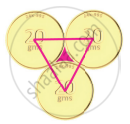Exercise 2.4 | Q 8. (i) | Page 73

Using Euler’s formula, find the unknowns.

 Faces Vertices Edges ? 6 14
Exercise 2.4 | Q 8. (ii) | Page 73

Using Euler’s formula, find the unknowns.

 Faces Vertices Edges 8 ? 10
Exercise 2.4 | Q 8. (iii) | Page 73

Using Euler’s formula, find the unknowns.

 Faces Verticles Edges 20 10 ?

## Chapter 2: Measurements

Exercise 2.1Exercise 2.2Exercise 2.3Exercise 2.4## Tamil Nadu Board Samacheer Kalvi solutions for Class 8th Mathematics Answers Guide chapter 2 - Measurements

Tamil Nadu Board Samacheer Kalvi solutions for Class 8th Mathematics Answers Guide chapter 2 (Measurements) include all questions with solution and detail explanation. This will clear students doubts about any question and improve application skills while preparing for board exams. The detailed, step-by-step solutions will help you understand the concepts better and clear your confusions, if any. Shaalaa.com has the Tamil Nadu Board of Secondary Education Class 8th Mathematics Answers Guide solutions in a manner that help students grasp basic concepts better and faster.

Further, we at Shaalaa.com provide such solutions so that students can prepare for written exams. Tamil Nadu Board Samacheer Kalvi textbook solutions can be a core help for self-study and acts as a perfect self-help guidance for students.

Concepts covered in Class 8th Mathematics Answers Guide chapter 2 Measurements are Arc, Segment, Sector, Central Angle and the Measure of an Arc, Length of an Arc, Area of the Sector, Perimeter of a Sector, Perimeter and Area of Combined Shapes, Three Dimensional Shapes, Faces, Edges and Vertices, Nets for Building 3-d Shapes - Cube, Cuboids, Cylinders, Cones, Pyramid, and Prism, Drawing Solids on a Flat Surface - Isometric Sketches, Cross Section of Solid Shapes, Measurements, Concept of Circle - Centre, Radius, Diameter, Arc, Sector, Chord, Segment, Semicircle, Circumference, Interior and Exterior, Concentric Circles, Viewing Different Sections of a Solid.

Using Tamil Nadu Board Samacheer Kalvi Class 8th solutions Measurements exercise by students are an easy way to prepare for the exams, as they involve solutions arranged chapter-wise also page wise. The questions involved in Tamil Nadu Board Samacheer Kalvi Solutions are important questions that can be asked in the final exam. Maximum students of Tamil Nadu Board of Secondary Education Class 8th prefer Tamil Nadu Board Samacheer Kalvi Textbook Solutions to score more in exam.

Get the free view of chapter 2 Measurements Class 8th extra questions for Class 8th Mathematics Answers Guide and can use Shaalaa.com to keep it handy for your exam preparation